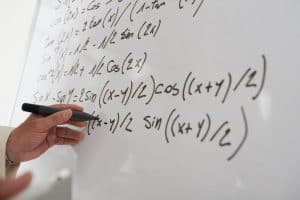## Algebra – What Is It?

Algebra, a branch of mathematics, involves the application of arithmetic operations and formal manipulations to abstract symbols rather than specific numbers. It encompasses various fields of mathematical research and finds practical applications in science, engineering, and mathematics as a whole.To utilize algebra effectively, one must understand the techniques for expressing and solving complex mathematical equations. These techniques involve transforming variables on one side of an equation and constants on the other side, as well as performing arithmetic operations such as addition, subtraction, multiplication, and division.

The origins of algebra can be traced back to ancient civilizations. The Babylonians and Greeks, known for their advancements in mathematics, including astronomy and navigation, played a significant role in its development. However, the term “algebra” itself came into common usage much later, during the 16th or 17th century.

When approaching algebraic expressions, the initial step is to identify and group similar variables on one side of the equation. Then, the other side of the equation is rewritten to include all these similar variables. Once all the terms are rewritten, basic arithmetic operations can be performed to solve the equation.

Commutative rules of addition and multiplication play a crucial role in algebra. The commutative rule of addition states that the sum of any two numbers is the same regardless of their order. Similarly, the commutative rule of multiplication asserts that the product of two numbers remains unchanged irrespective of their order. These rules are useful for comparing integers, verifying divisibility, and ensuring the equality of two different integers.

In commutative algebra, certain rings of integers possess specific properties that make them ideal for study. These rings have applications in various branches of mathematics, including number theory, geometric topology, and algebraic geometry. Examples of commutative rings include algebraic integers, polynomial rings, and rings of positive numbers. The study of commutative rings and their ideals forms the foundation for other areas of pure mathematics, such as differential topology, invariant theory, order theory, and general topology.

Associativity is another property of addition and multiplication that plays a role in determining inverse elements. The inverse of a number “a” is represented as “-a,” while the inverse of “a” multiplied by itself is represented as “a-1.”

Identifying identities is an important concept in algebra, particularly when considering the commutative rules of addition and multiplication. An identity element refers to a single number that, when combined with another number, does not change its value. Using this property, the identity element can be found for each element in a complex number system. Common examples include the identity of a complex number and the identity of the first element in a complex sequence.

Algebra provides a powerful framework for understanding and solving mathematical problems. By applying algebraic techniques, one can express and solve complex equations, explore commutative rules, study commutative rings, determine inverse elements, and identify identities. This branch of mathematics continues to shape our understanding of the world around us and contributes to advancements in various fields of study.